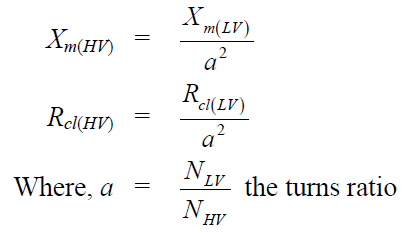# Open circuit and Short circuit test on Transformers

Open circuit and short circuit tests are performed on a transformer to determine the following:

• equivalent circuit of transformer

• voltage regulation of transformer

• Efficiency of the transformer. The power required for these open circuit test and short circuit test on transformer is equal to the power loss occurring in the transformer.

## Open circuit or No load test:

For O.C. test rated voltage should be applied to the transformer and a wattmeter to measure loss (power) and an ammeter to measure no load current should be connected as shown and the secondary should be left open.

The voltmeter, ammeter and the wattmeter readings are taken and suppose they are V0, I0 and W0 respectively. The winding where the supply is connected becomes the primary and the other the secondary. Since the rated voltage is to be applied, it is safer from an operation point of view to use LV winding as the primary winding.

Also, the meters required for conducting the O.C. test will have low ratings and are easily available in testing laboratories. So we use LV winding as the primary winding.

The wattmeter will record the core loss as well as the LV winding copper loss. But the winding copper loss is very small compared to the core loss as the flux in the core is rated. In fact, this approximation is built-in in the approximate equivalent circuit of the transformer referred to the primary side which is LV side in this case.## Short circuit test:

Short circuit test is generally carried out by energizing the HV side with LV side shorted. Voltage applied is such that the rated current flows in the windings.

Here the shunt branch is omitted as the current drawn by the shunt J0 is negligibly small as compared to full load current and when short circuit is carried out the requirement is that the supply voltage should be such that rated current flows in the two windings.

The short circuit test is most conveniently made by short-circuiting the LT winding, for this means that the current to be measured will be the moderate amount corresponding to full load on the HT side and at the same time the voltage to be supplied will be only a small fraction of the normal voltage of the HT winding, therefore, within the range of meters normally available in the testing laboratories.

The primary voltage is gradually applied till the rated current flows in the winding. Since, the applied voltage is very small ( may be of the order of 5 − 8%), the magnetizing branch can now be eliminated from the equivalent circuit. Suppose the readings are Vsc, Isc and Wsc.If Vsc is the applied voltage to circulate the rated current (I’2 ) on short circuit, and Pc is the power input to the transformer then,